# 8.7 Parametric equations: graphs  (Page 3/4)

 Page 3 / 4

Access the following online resource for additional instruction and practice with graphs of parametric equations.

## Key concepts

• When there is a third variable, a third parameter on which $\text{\hspace{0.17em}}x\text{\hspace{0.17em}}$ and $\text{\hspace{0.17em}}y\text{\hspace{0.17em}}$ depend, parametric equations can be used.
• To graph parametric equations by plotting points, make a table with three columns labeled $\text{\hspace{0.17em}}t,x\left(t\right),\text{\hspace{0.17em}}$ and $\text{\hspace{0.17em}}y\left(t\right).\text{\hspace{0.17em}}$ Choose values for $\text{\hspace{0.17em}}t\text{\hspace{0.17em}}$ in increasing order. Plot the last two columns for $\text{\hspace{0.17em}}x\text{\hspace{0.17em}}$ and $\text{\hspace{0.17em}}y.\text{\hspace{0.17em}}$ See [link] and [link] .
• When graphing a parametric curve by plotting points, note the associated t -values and show arrows on the graph indicating the orientation of the curve. See [link] and [link] .
• Parametric equations allow the direction or the orientation of the curve to be shown on the graph. Equations that are not functions can be graphed and used in many applications involving motion. See [link] .
• Projectile motion depends on two parametric equations: $\text{\hspace{0.17em}}x=\left({v}_{0}\mathrm{cos}\text{\hspace{0.17em}}\theta \right)t\text{\hspace{0.17em}}$ and $\text{\hspace{0.17em}}y=-16{t}^{2}+\left({v}_{0}\mathrm{sin}\text{\hspace{0.17em}}\theta \right)t+h.\text{\hspace{0.17em}}$ Initial velocity is symbolized as $\text{\hspace{0.17em}}{v}_{0}.\text{\hspace{0.17em}}\theta$ represents the initial angle of the object when thrown, and $\text{\hspace{0.17em}}h\text{\hspace{0.17em}}$ represents the height at which the object is propelled.

## Verbal

What are two methods used to graph parametric equations?

plotting points with the orientation arrow and a graphing calculator

What is one difference in point-plotting parametric equations compared to Cartesian equations?

Why are some graphs drawn with arrows?

The arrows show the orientation, the direction of motion according to increasing values of $\text{\hspace{0.17em}}t.$

Name a few common types of graphs of parametric equations.

Why are parametric graphs important in understanding projectile motion?

The parametric equations show the different vertical and horizontal motions over time.

## Graphical

For the following exercises, graph each set of parametric equations by making a table of values. Include the orientation on the graph.

$\left\{\begin{array}{l}x\left(t\right)=t\hfill \\ y\left(t\right)={t}^{2}-1\hfill \end{array}$

 $t$ $x$ $y$ $-3$ $-2$ $-1$ $0$ $1$ $2$ $3$

$\left\{\begin{array}{l}x\left(t\right)=t-1\hfill \\ y\left(t\right)={t}^{2}\hfill \end{array}$

 $t$ $-3$ $-2$ $-1$ $0$ $1$ $2$ $x$ $y$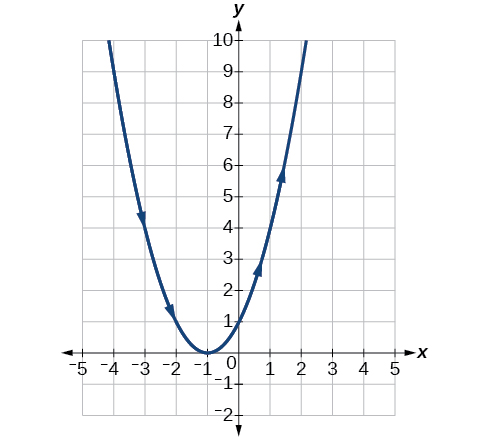$\left\{\begin{array}{l}x\left(t\right)=2+t\hfill \\ y\left(t\right)=3-2t\hfill \end{array}$

 $t$ $-2$ $-1$ $0$ $1$ $2$ $3$ $x$ $y$

$\left\{\begin{array}{l}x\left(t\right)=-2-2t\hfill \\ y\left(t\right)=3+t\hfill \end{array}$

 $t$ $-3$ $-2$ $-1$ $0$ $1$ $x$ $y$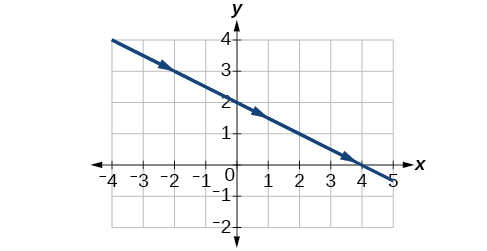$\left\{\begin{array}{l}x\left(t\right)={t}^{3}\hfill \\ y\left(t\right)=t+2\hfill \end{array}$

 $t$ $-2$ $-1$ $0$ $1$ $2$ $x$ $y$

$\left\{\begin{array}{l}x\left(t\right)={t}^{2}\hfill \\ y\left(t\right)=t+3\hfill \end{array}$

 $t$ $-2$ $-1$ $0$ $1$ $2$ $x$ $y$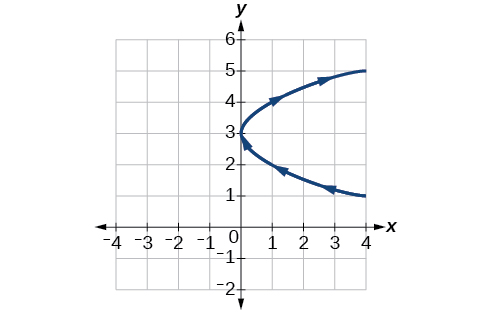For the following exercises, sketch the curve and include the orientation.

$\left\{\begin{array}{l}x\left(t\right)=t\\ y\left(t\right)=\sqrt{t}\end{array}$

$\left\{\begin{array}{l}x\left(t\right)=-\text{\hspace{0.17em}}\sqrt{t}\\ y\left(t\right)=t\end{array}$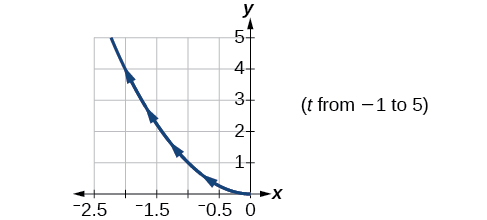$\left\{\begin{array}{l}x\left(t\right)=5-|t|\\ y\left(t\right)=t+2\end{array}$

$\left\{\begin{array}{l}x\left(t\right)=-t+2\\ y\left(t\right)=5-|t|\end{array}$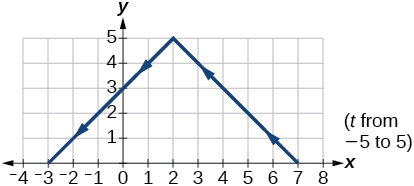$\left\{\begin{array}{l}x\left(t\right)=4\text{sin}\text{\hspace{0.17em}}t\hfill \\ y\left(t\right)=2\mathrm{cos}\text{\hspace{0.17em}}t\hfill \end{array}$

$\left\{\begin{array}{l}x\left(t\right)=2\text{sin}\text{\hspace{0.17em}}t\hfill \\ y\left(t\right)=4\text{cos}\text{\hspace{0.17em}}t\hfill \end{array}$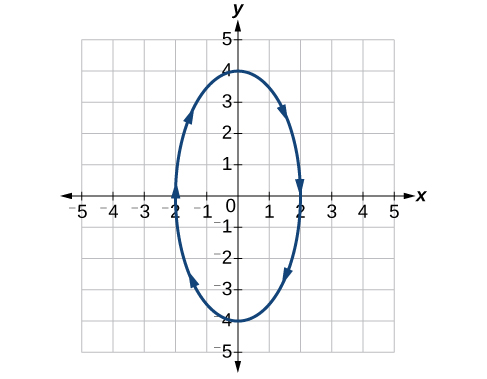$\left\{\begin{array}{l}x\left(t\right)=3{\mathrm{cos}}^{2}t\\ y\left(t\right)=-3\mathrm{sin}\text{\hspace{0.17em}}t\end{array}$

$\left\{\begin{array}{l}x\left(t\right)=3{\mathrm{cos}}^{2}t\\ y\left(t\right)=-3{\mathrm{sin}}^{2}t\end{array}$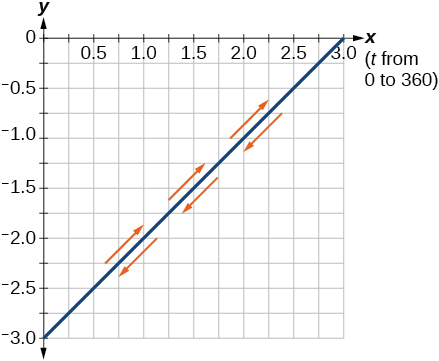$\left\{\begin{array}{l}x\left(t\right)=\mathrm{sec}\text{\hspace{0.17em}}t\\ y\left(t\right)=\mathrm{tan}\text{\hspace{0.17em}}t\end{array}$

$\left\{\begin{array}{l}x\left(t\right)=\mathrm{sec}\text{\hspace{0.17em}}t\\ y\left(t\right)={\mathrm{tan}}^{2}t\end{array}$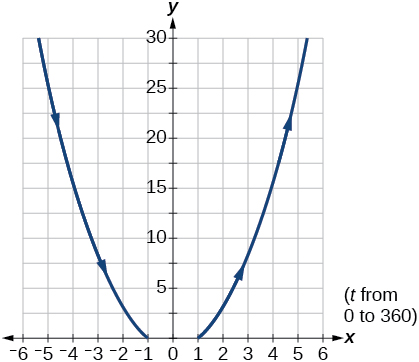$\left\{\begin{array}{l}x\left(t\right)=\frac{1}{{e}^{2t}}\\ y\left(t\right)={e}^{-\text{\hspace{0.17em}}t}\end{array}$

For the following exercises, graph the equation and include the orientation. Then, write the Cartesian equation.

$\left\{\begin{array}{l}x\left(t\right)=t-1\hfill \\ y\left(t\right)=-{t}^{2}\hfill \end{array}$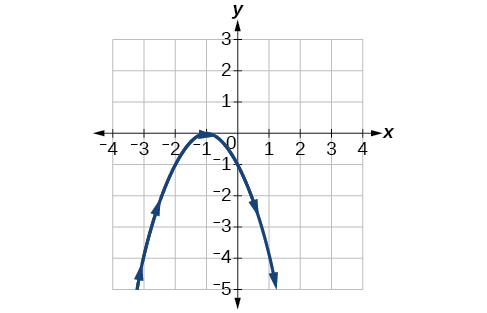$\left\{\begin{array}{l}x\left(t\right)={t}^{3}\hfill \\ y\left(t\right)=t+3\hfill \end{array}$

$\left\{\begin{array}{l}x\left(t\right)=2\mathrm{cos}\text{\hspace{0.17em}}t\\ y\left(t\right)=-\mathrm{sin}\text{\hspace{0.17em}}t\end{array}$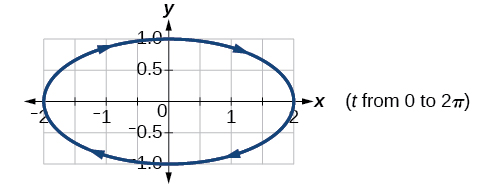$\left\{\begin{array}{l}x\left(t\right)=7\mathrm{cos}\text{\hspace{0.17em}}t\\ y\left(t\right)=7\mathrm{sin}\text{\hspace{0.17em}}t\end{array}$

$\left\{\begin{array}{l}x\left(t\right)={e}^{2t}\\ y\left(t\right)=-{e}^{\text{\hspace{0.17em}}t}\end{array}$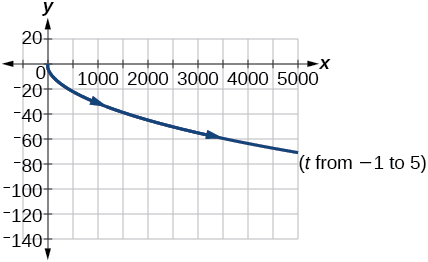For the following exercises, graph the equation and include the orientation.

$x={t}^{2},\text{\hspace{0.17em}}y\text{\hspace{0.17em}}=\text{\hspace{0.17em}}3t,\text{\hspace{0.17em}}0\le t\le 5$

$x=2t,\text{\hspace{0.17em}}y\text{\hspace{0.17em}}=\text{\hspace{0.17em}}\text{\hspace{0.17em}}{t}^{2},\text{\hspace{0.17em}}-5\le t\le 5$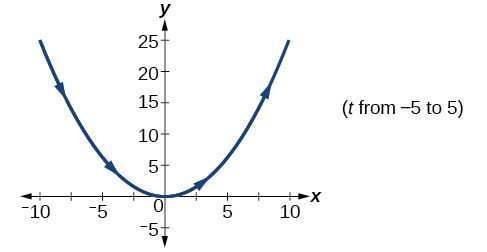$x=t,\text{\hspace{0.17em}}y=\sqrt{25-{t}^{2}},\text{\hspace{0.17em}}0

$x\left(t\right)=-t,y\left(t\right)=\sqrt{t},\text{\hspace{0.17em}}t\ge 0$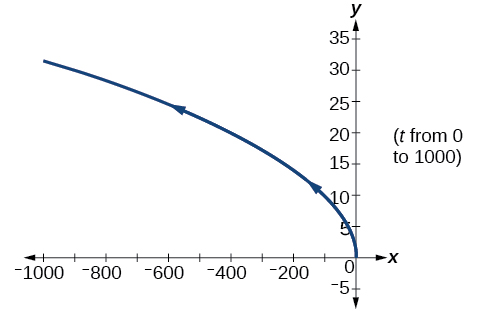$x=-2\mathrm{cos}\text{\hspace{0.17em}}t,\text{\hspace{0.17em}}y=6\text{\hspace{0.17em}}\mathrm{sin}\text{\hspace{0.17em}}t,\text{\hspace{0.17em}}0\le t\le \pi$

$x=-\mathrm{sec}\text{\hspace{0.17em}}t,\text{\hspace{0.17em}}y=\mathrm{tan}\text{\hspace{0.17em}}t,\text{\hspace{0.17em}}-\frac{\text{\hspace{0.17em}}\pi }{2}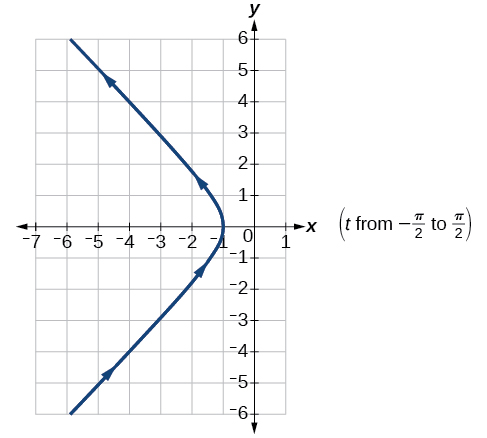For the following exercises, use the parametric equations for integers a and b :

$\begin{array}{l}x\left(t\right)=a\mathrm{cos}\left(\left(a+b\right)t\right)\\ y\left(t\right)=a\mathrm{cos}\left(\left(a-b\right)t\right)\end{array}$

Graph on the domain $\text{\hspace{0.17em}}\left[-\pi ,0\right],\text{\hspace{0.17em}}$ where $\text{\hspace{0.17em}}a=2\text{\hspace{0.17em}}$ and $\text{\hspace{0.17em}}b=1,\text{\hspace{0.17em}}$ and include the orientation.

Graph on the domain $\text{\hspace{0.17em}}\left[-\pi ,0\right],\text{\hspace{0.17em}}$ where $\text{\hspace{0.17em}}a=3\text{\hspace{0.17em}}$ and $\text{\hspace{0.17em}}b=2$ , and include the orientation.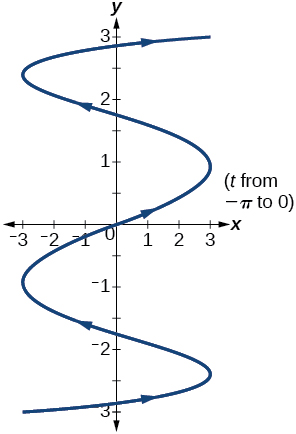Graph on the domain $\text{\hspace{0.17em}}\left[-\pi ,0\right],\text{\hspace{0.17em}}$ where $\text{\hspace{0.17em}}a=4\text{\hspace{0.17em}}$ and $\text{\hspace{0.17em}}b=3$ , and include the orientation.

can you not take the square root of a negative number
No because a negative times a negative is a positive. No matter what you do you can never multiply the same number by itself and end with a negative
lurverkitten
Actually you can. you get what's called an Imaginary number denoted by i which is represented on the complex plane. The reply above would be correct if we were still confined to the "real" number line.
Liam
Suppose P= {-3,1,3} Q={-3,-2-1} and R= {-2,2,3}.what is the intersection
can I get some pretty basic questions
In what way does set notation relate to function notation
Ama
is precalculus needed to take caculus
It depends on what you already know. Just test yourself with some precalculus questions. If you find them easy, you're good to go.
Spiro
the solution doesn't seem right for this problem
what is the domain of f(x)=x-4/x^2-2x-15 then
x is different from -5&3
Seid
All real x except 5 and - 3
Spiro
***youtu.be/ESxOXfh2Poc
Loree
how to prroved cos⁴x-sin⁴x= cos²x-sin²x are equal
Don't think that you can.
Elliott
By using some imaginary no.
Tanmay
how do you provided cos⁴x-sin⁴x = cos²x-sin²x are equal
What are the question marks for?
Elliott
Someone should please solve it for me Add 2over ×+3 +y-4 over 5 simplify (×+a)with square root of two -×root 2 all over a multiply 1over ×-y{(×-y)(×+y)} over ×y
For the first question, I got (3y-2)/15 Second one, I got Root 2 Third one, I got 1/(y to the fourth power) I dont if it's right cause I can barely understand the question.
Is under distribute property, inverse function, algebra and addition and multiplication function; so is a combined question
Abena
find the equation of the line if m=3, and b=-2
graph the following linear equation using intercepts method. 2x+y=4
Ashley
how
Wargod
what?
John
ok, one moment
UriEl
how do I post your graph for you?
UriEl
it won't let me send an image?
UriEl
also for the first one... y=mx+b so.... y=3x-2
UriEl
y=mx+b you were already given the 'm' and 'b'. so.. y=3x-2
Tommy
Please were did you get y=mx+b from
Abena
y=mx+b is the formula of a straight line. where m = the slope & b = where the line crosses the y-axis. In this case, being that the "m" and "b", are given, all you have to do is plug them into the formula to complete the equation.
Tommy
thanks Tommy
Nimo
0=3x-2 2=3x x=3/2 then . y=3/2X-2 I think
Given
co ordinates for x x=0,(-2,0) x=1,(1,1) x=2,(2,4)
neil
"7"has an open circle and "10"has a filled in circle who can I have a set builder notation
Where do the rays point?
Spiro
x=-b+_Гb2-(4ac) ______________ 2a
I've run into this: x = r*cos(angle1 + angle2) Which expands to: x = r(cos(angle1)*cos(angle2) - sin(angle1)*sin(angle2)) The r value confuses me here, because distributing it makes: (r*cos(angle2))(cos(angle1) - (r*sin(angle2))(sin(angle1)) How does this make sense? Why does the r distribute once
so good
abdikarin
this is an identity when 2 adding two angles within a cosine. it's called the cosine sum formula. there is also a different formula when cosine has an angle minus another angle it's called the sum and difference formulas and they are under any list of trig identities
strategies to form the general term
carlmark
consider r(a+b) = ra + rb. The a and b are the trig identity.
Mike
How can you tell what type of parent function a graph is ?
generally by how the graph looks and understanding what the base parent functions look like and perform on a graph
William
if you have a graphed line, you can have an idea by how the directions of the line turns, i.e. negative, positive, zero
William
y=x will obviously be a straight line with a zero slope
William
y=x^2 will have a parabolic line opening to positive infinity on both sides of the y axis vice versa with y=-x^2 you'll have both ends of the parabolic line pointing downward heading to negative infinity on both sides of the y axis
William
y=x will be a straight line, but it will have a slope of one. Remember, if y=1 then x=1, so for every unit you rise you move over positively one unit. To get a straight line with a slope of 0, set y=1 or any integer.
Aaron
yes, correction on my end, I meant slope of 1 instead of slope of 0
William
what is f(x)=
I don't understand
Joe
Typically a function 'f' will take 'x' as input, and produce 'y' as output. As 'f(x)=y'. According to Google, "The range of a function is the complete set of all possible resulting values of the dependent variable (y, usually), after we have substituted the domain."
Thomas
Sorry, I don't know where the "Â"s came from. They shouldn't be there. Just ignore them. :-)
Thomas
Darius
Thanks.
Thomas
Â
Thomas
It is the Â that should not be there. It doesn't seem to show if encloses in quotation marks. "Â" or 'Â' ... Â
Thomas
Now it shows, go figure?
ThomasByByBy Bonnie HurstBy OpenStaxBy Richley CrapoBy Vanessa SoledadBy Mistry BhaveshBy Caitlyn GobbleBy OpenStaxBy Eddie UnverzagtBy OpenStaxBy OpenStax